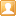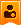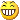##帐号 自动登录 找回密码 密码 注册

 搜索

# 使用Mircoduino UM220III gps模块自动校准RTC PCF8563制作高精度北京时间时钟发表于 2016-9-6 23:07:54 | 显示全部楼层 |阅读模式
 Microduino mcookie的教程里只有手动设置RTC来制作时钟，本帖介绍一下，用UM220III gps模块自动校准RTC PCF8563，并且再用RTC PCF8563制作高精度北京时间时钟。 有关UM220III gps模块得到时间和日期的办法帖子《Microduino-UM220iiiN-GPS模块制作时钟》已经介绍过了，gps的日期转化成北京日期的办法switch（）来实现，这样阅读起来也比较方便。就是每天24点，根据月份判断明天的日期。 switch(gps.Month) { case 1:   if(GPS_Hour_Tmp >= 24)   {                       gps.Day += 1;     if(gps.Day > 31)     {       gps.Day -= 31;       gps.Month += 1;     }   }   break; case 2:   if(GPS_Hour_Tmp >= 24)   {                       gps.Day += 1;     if( LEAP_YEAR = 1)     {       if(gps.Day > 29)       {         gps.Day -= 29;         gps.Month += 1;         }     }     else     {       if(gps.Day > 28)       {         gps.Day -= 28;         gps.Month += 1;       }     }   }   break;       case 3:   if(GPS_Hour_Tmp >= 24)   {                          gps.Day += 1;     if(gps.Day > 31)     {       gps.Day -= 31;       gps.Month += 1;     }   }   break; case 4:   if(GPS_Hour_Tmp >= 24)   {                       gps.Day += 1;     if(gps.Day > 30)     {       gps.Day -= 30;       gps.Month += 1;     }   }   break; case 5:   if(GPS_Hour_Tmp >= 24)   {                    gps.Day += 1;     if(gps.Day > 31)     {       gps.Day -= 31;       gps.Month += 1;     }   }   break; case 6:   if(GPS_Hour_Tmp >= 24)   {                      gps.Day += 1;     if(gps.Day > 30)     {       gps.Day -= 30;       gps.Month += 1;     }   }   break; case 7:   if(GPS_Hour_Tmp >= 24)   {                    gps.Day += 1;     if(gps.Day > 31)     {       gps.Day -= 31;       gps.Month += 1;     }   }   break; case 8:   if(GPS_Hour_Tmp >= 24)   {                       gps.Day += 1;     if(gps.Day > 31)     {       gps.Day -= 31;       gps.Month += 1;     }   }   break; case 9:   if(GPS_Hour_Tmp >= 24)   {                       gps.Day += 1;     if(gps.Day > 30)     {       gps.Day -= 30;       gps.Month += 1;     }   }   break; case 10:   if(GPS_Hour_Tmp >= 24)   {                          gps.Day += 1;     if(gps.Day > 31)     {       gps.Day -= 31;       gps.Month += 1;     }   }   break; case 11:   if(GPS_Hour_Tmp >= 24)   {                         gps.Day += 1;     if(gps.Day > 30)     {       gps.Day -= 30;       gps.Month += 1;     }   } } break; case 12:   if(GPS_Hour_Tmp >= 24)   {                          gps.Day += 1;     if(gps.Day > 31)     {       gps.Day -= 31;       gps.Month = 1;       gps.Year += 1;     }   }   break; default:   break; 复制代码 RTC PCF8563设置日期的函数是 rtc.setDate();参数包括day, weekday, month, century(1=1900, 0=2000), year(0-99)。其中year只要后面两位，所以在截取gps的年份时对"\$GNZDA"取了26至28位。难在对星期的计算上，这里我是用了基姆拉尔森计算公式。把一月和二月看成是上一年的十三月和十四月。年月日转化成星期几的公式是 weekday= (day+2*month+3*(month+1)/5+year+year/4-year/100+year/400+1)%7 。计算出来的“0”代表星期一，“6”代表星期天，依此类推。 if(gps.Month ==1||gps.Month ==2) {              gps.Month=(gps.Month==1?13:14);              gps.Year--;              }              int Weekday=(gps.Day+2*gps.Month +3*(gps.Month +1)/5+gps.Year+gps.Year/4-gps.Year/100+gps.Year/400)%7;                        把Microduino um220III , coreUSB 和 mcookie RTC PCF8563叠加在一起，用数据线和电脑的USB口连接，用Arduino IDE烧写程序。 程序分享在github地址： 在串口看到数据已经被改写，和计算机右下角的时间相同，那么校准RTC的任务已经完成。 接下来要使用mcookie的模块来做时钟，用到的有coreUSB,RTC,OLED,BM和电池组，以及底板。用Arduino IDE烧写程序。程序分享到github地址： 时间的精度和电波表不相上下，那比手动对时精度高多了。最后的显示效果：

### 本帖子中包含更多资源

x发表于 2016-9-7 11:23:54 | 显示全部楼层
 那两节1860很是，威武发表于 2016-9-7 18:06:25 | 显示全部楼层
 蹲蹲兄，转换东八区的时间，有现成库，你可以参考一下。https://github.com/jcw/rtclib //提取时间     i_hour=GPS.hour;     i_minute=GPS.minute;     i_second=GPS.seconds;     i_year=GPS.year;     i_month=GPS.month;     i_day=GPS.day;     DateTime dt1 (i_year,i_month, i_day, i_hour, i_minute, i_second);     DateTime dt2=dt1.get()+3600*8;  //8 hour later （东八区）     i_year=dt2.year();//将补偿8小时的时间重新计算年月日时     i_month=dt2.month();     i_day=dt2.day();     i_hour=dt2.hour();复制代码楼主| 发表于 2016-9-7 19:42:45 | 显示全部楼层
 沧海笑1122 发表于 2016-9-7 18:06 蹲蹲兄，转换东八区的时间，有现成库，你可以参考一下。https://github.com/jcw/rtclib 谢谢指教，一起学习进步。楼主| 发表于 2016-9-9 20:35:11 | 显示全部楼层
 wing 发表于 2016-9-7 11:23 那两节1860很是，威武 是10440。谢谢指导。发表于 2016-9-10 10:05:47 | 显示全部楼层
 哦哦,小白了锂点在我看来都很高大上楼主| 发表于 2016-9-10 22:39:49 | 显示全部楼层
 wing 发表于 2016-9-10 10:05 哦哦,小白了 锂点在我看来都很高大上 谢谢你的指导。

 本版积分规则 需要先绑定手机号 回帖后跳转到最后一页

GMT+8, 2023-10-1 09:44 , Processed in 0.045930 second(s), 20 queries .